Скачать презентацию Machine Learning for Online Decision Making applications

2e548acda64301f038555799c1ca000e.ppt

• Количество слайдов: 20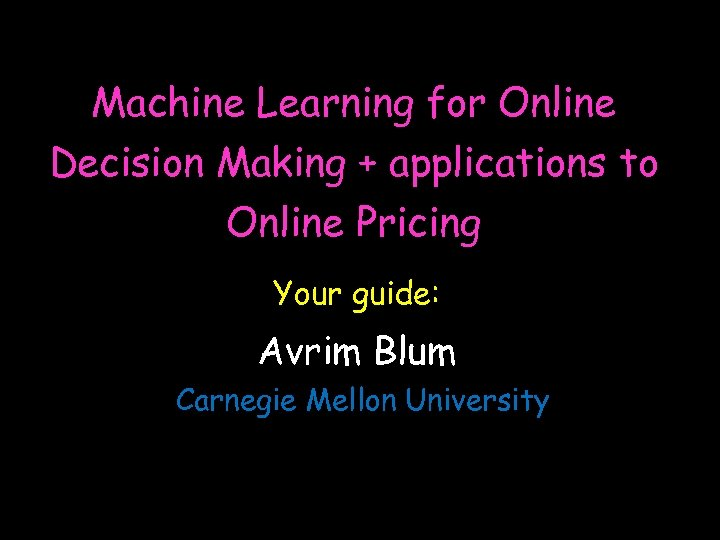Machine Learning for Online Decision Making + applications to Online Pricing Your guide: Avrim Blum Carnegie Mellon University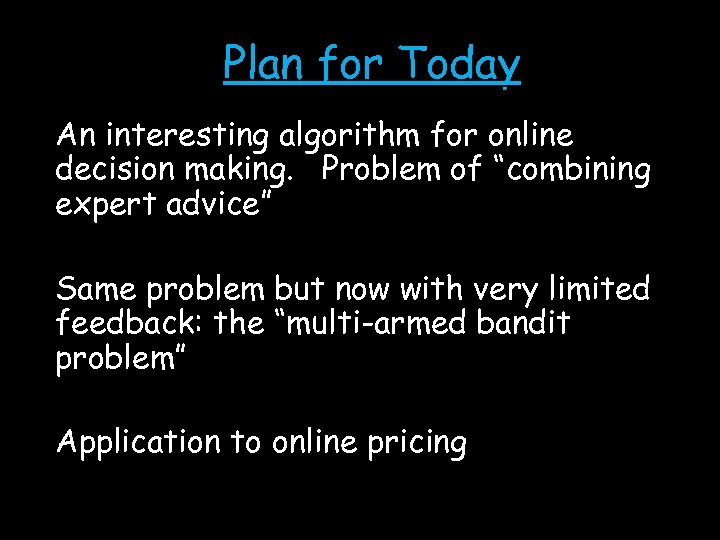Plan for Today An interesting algorithm for online decision making. Problem of “combining expert advice” Same problem but now with very limited feedback: the “multi-armed bandit problem” Application to online pricing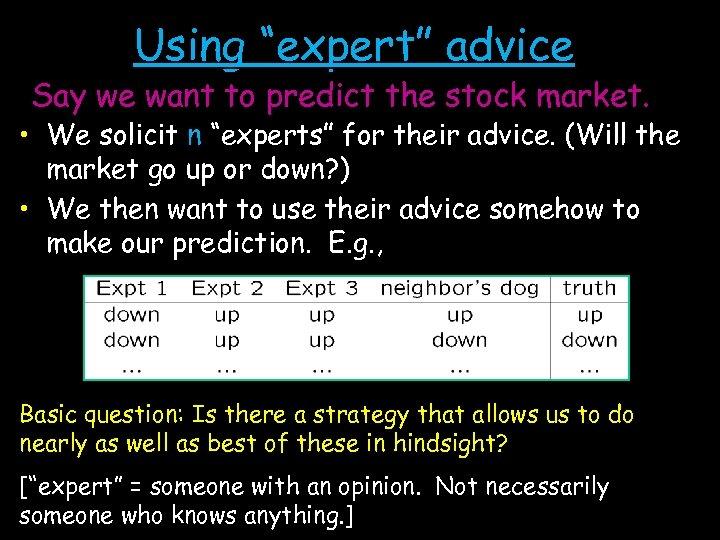Using “expert” advice Say we want to predict the stock market. • We solicit n “experts” for their advice. (Will the market go up or down? ) • We then want to use their advice somehow to make our prediction. E. g. , Basic question: Is there a strategy that allows us to do nearly as well as best of these in hindsight? [“expert” = someone with an opinion. Not necessarily someone who knows anything. ]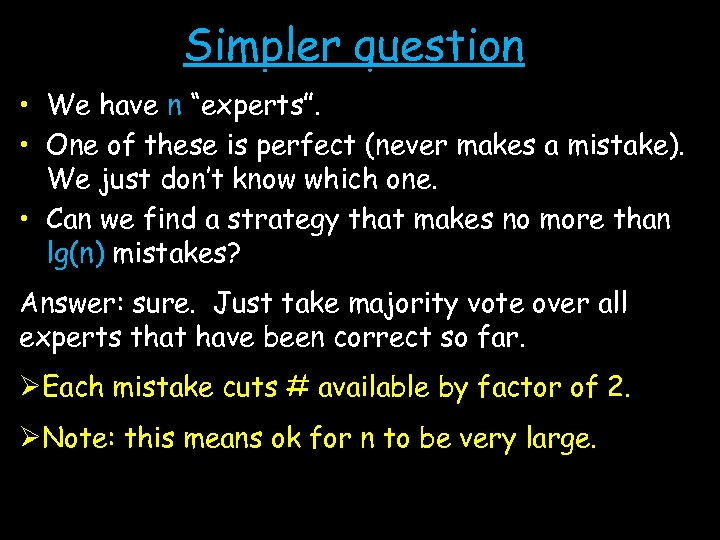Simpler question • We have n “experts”. • One of these is perfect (never makes a mistake). We just don’t know which one. • Can we find a strategy that makes no more than lg(n) mistakes? Answer: sure. Just take majority vote over all experts that have been correct so far. ØEach mistake cuts # available by factor of 2. ØNote: this means ok for n to be very large.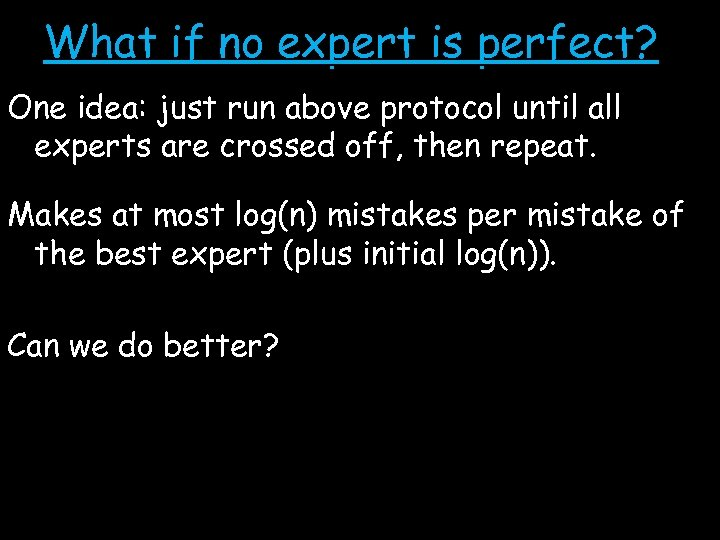What if no expert is perfect? One idea: just run above protocol until all experts are crossed off, then repeat. Makes at most log(n) mistakes per mistake of the best expert (plus initial log(n)). Can we do better?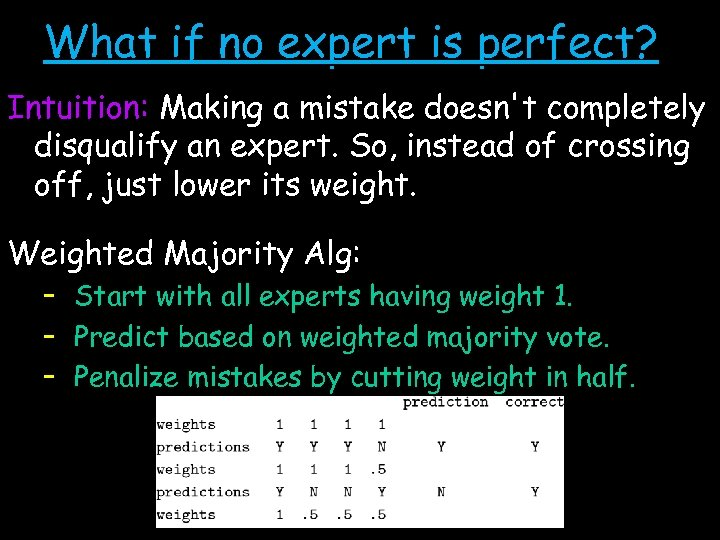What if no expert is perfect? Intuition: Making a mistake doesn't completely disqualify an expert. So, instead of crossing off, just lower its weight. Weighted Majority Alg: – Start with all experts having weight 1. – Predict based on weighted majority vote. – Penalize mistakes by cutting weight in half.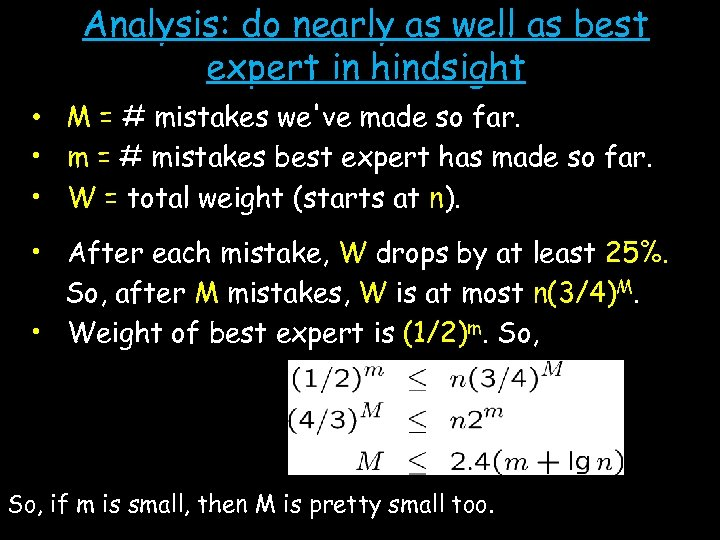Analysis: do nearly as well as best expert in hindsight • M = # mistakes we've made so far. • m = # mistakes best expert has made so far. • W = total weight (starts at n). • After each mistake, W drops by at least 25%. So, after M mistakes, W is at most n(3/4)M. • Weight of best expert is (1/2)m. So, if m is small, then M is pretty small too.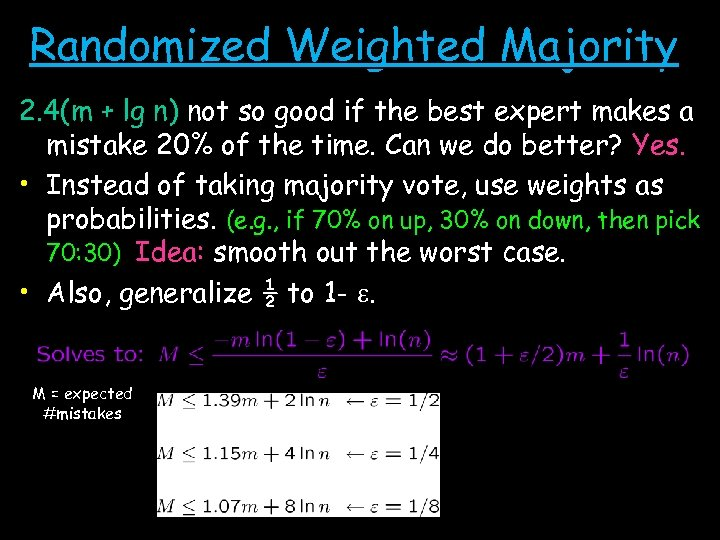Randomized Weighted Majority 2. 4(m + lg n) not so good if the best expert makes a mistake 20% of the time. Can we do better? Yes. • Instead of taking majority vote, use weights as probabilities. (e. g. , if 70% on up, 30% on down, then pick 70: 30) Idea: smooth out the worst case. • Also, generalize ½ to 1 - e. M = expected #mistakes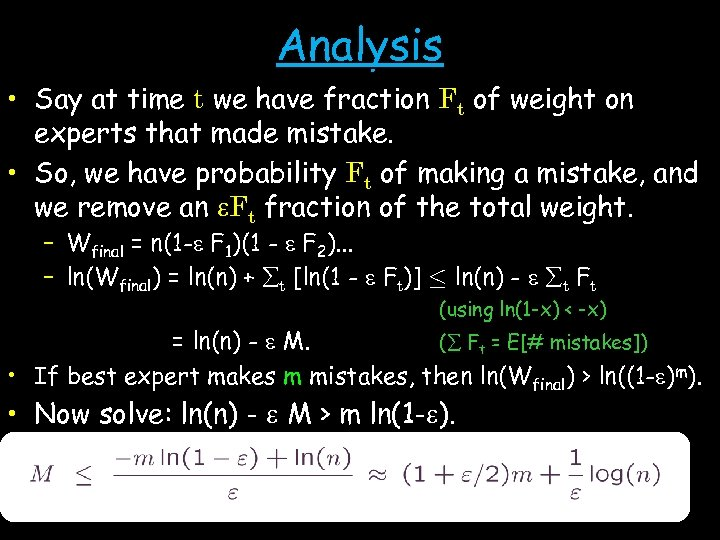Analysis • Say at time t we have fraction Ft of weight on experts that made mistake. • So, we have probability Ft of making a mistake, and we remove an e. Ft fraction of the total weight. – Wfinal = n(1 -e F 1)(1 - e F 2). . . – ln(Wfinal) = ln(n) + t [ln(1 - e Ft)] · ln(n) - e t Ft (using ln(1 -x) < -x) = ln(n) - e M. ( Ft = E[# mistakes]) • If best expert makes m mistakes, then ln(Wfinal) > ln((1 -e)m). • Now solve: ln(n) - e M > m ln(1 -e).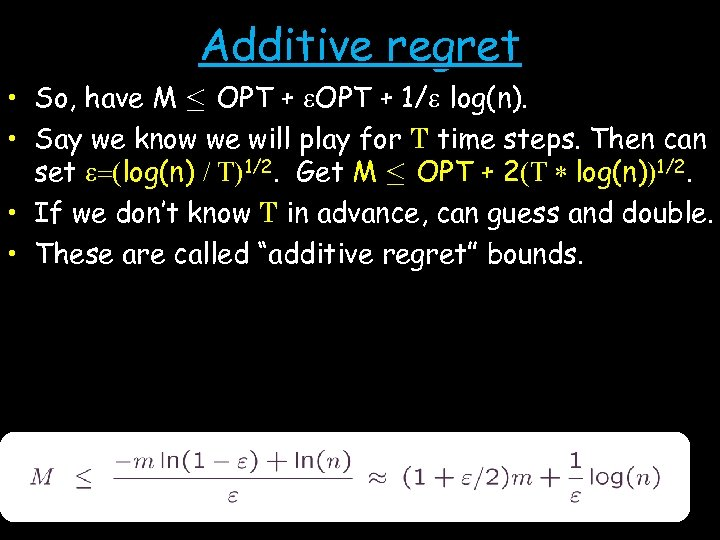Additive regret • So, have M · OPT + e. OPT + 1/e log(n). • Say we know we will play for T time steps. Then can set e=(log(n) / T)1/2. Get M · OPT + 2(T * log(n))1/2. • If we don’t know T in advance, can guess and double. • These are called “additive regret” bounds.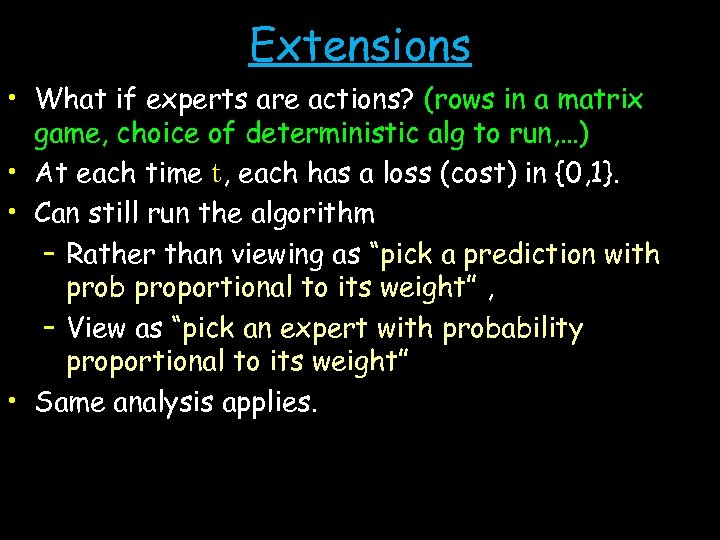Extensions • What if experts are actions? (rows in a matrix game, choice of deterministic alg to run, …) • At each time t, each has a loss (cost) in {0, 1}. • Can still run the algorithm – Rather than viewing as “pick a prediction with prob proportional to its weight” , – View as “pick an expert with probability proportional to its weight” • Same analysis applies.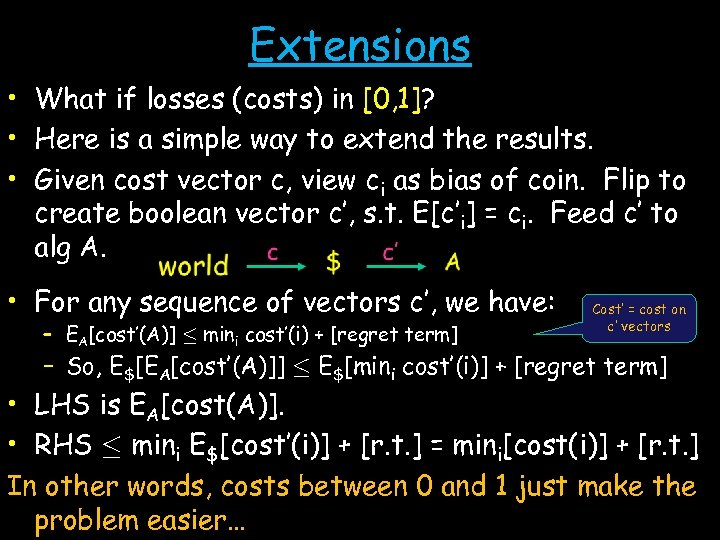Extensions • What if losses (costs) in [0, 1]? • Here is a simple way to extend the results. • Given cost vector c, view ci as bias of coin. Flip to create boolean vector c’, s. t. E[c’i] = ci. Feed c’ to alg A. • For any sequence of vectors c’, we have: – EA[cost’(A)] · mini cost’(i) + [regret term] Cost’ = cost on c’ vectors – So, E\$[EA[cost’(A)]] · E\$[mini cost’(i)] + [regret term] • LHS is EA[cost(A)]. • RHS · mini E\$[cost’(i)] + [r. t. ] = mini[cost(i)] + [r. t. ] In other words, costs between 0 and 1 just make the problem easier…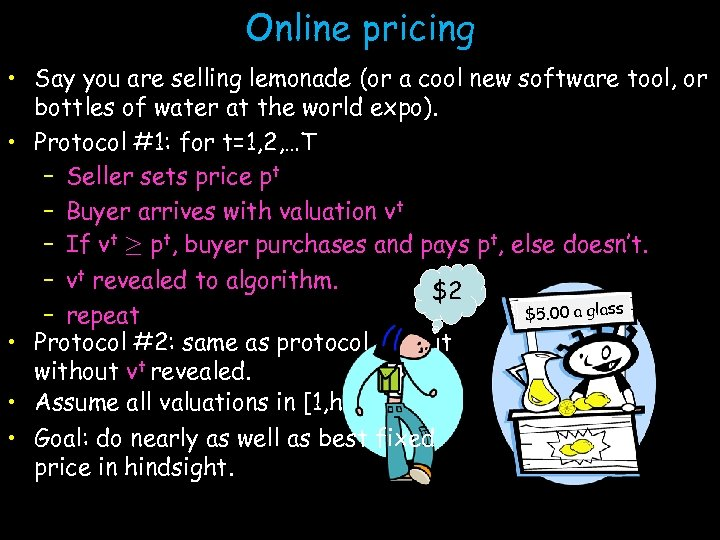Online pricing • Say you are selling lemonade (or a cool new software tool, or bottles of water at the world expo). • Protocol #1: for t=1, 2, …T – Seller sets price pt – Buyer arrives with valuation vt – If vt ¸ pt, buyer purchases and pays pt, else doesn’t. – vt revealed to algorithm. \$2 las \$5000 aagglass \$5. 0 – repeat • Protocol #2: same as protocol #1 but without vt revealed. • Assume all valuations in [1, h] • Goal: do nearly as well as best fixed price in hindsight.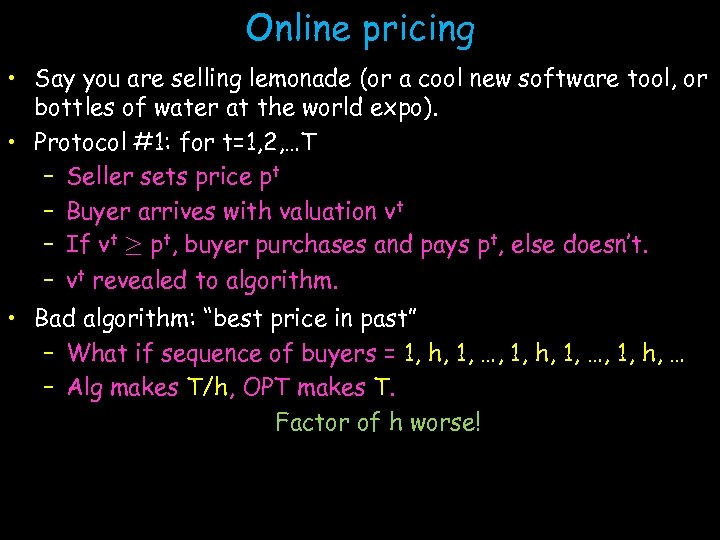Online pricing • Say you are selling lemonade (or a cool new software tool, or bottles of water at the world expo). • Protocol #1: for t=1, 2, …T – Seller sets price pt – Buyer arrives with valuation vt – If vt ¸ pt, buyer purchases and pays pt, else doesn’t. – vt revealed to algorithm. • Bad algorithm: “best price in past” – What if sequence of buyers = 1, h, 1, …, 1, h, … – Alg makes T/h, OPT makes T. Factor of h worse!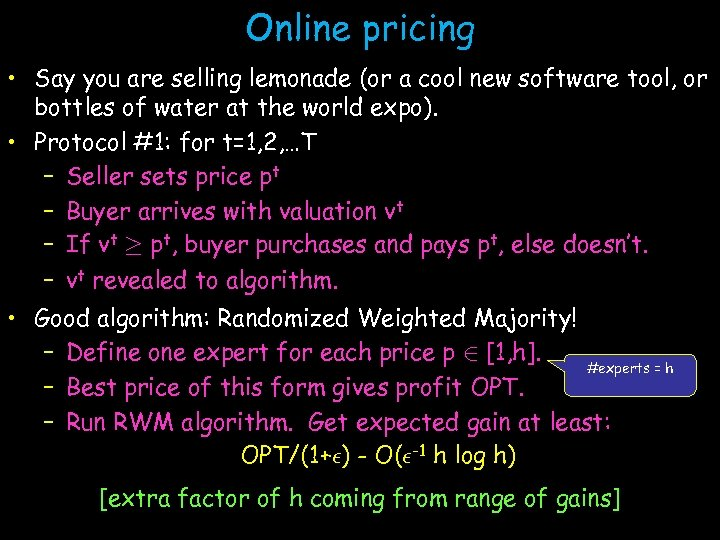Online pricing • Say you are selling lemonade (or a cool new software tool, or bottles of water at the world expo). • Protocol #1: for t=1, 2, …T – Seller sets price pt – Buyer arrives with valuation vt – If vt ¸ pt, buyer purchases and pays pt, else doesn’t. – vt revealed to algorithm. • Good algorithm: Randomized Weighted Majority! – Define one expert for each price p 2 [1, h]. #experts = h – Best price of this form gives profit OPT. – Run RWM algorithm. Get expected gain at least: OPT/(1+²) - O(²-1 h log h) [extra factor of h coming from range of gains]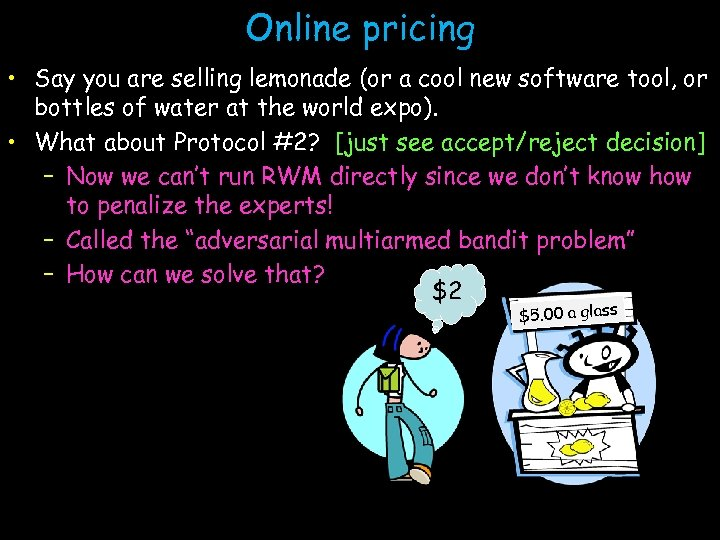Online pricing • Say you are selling lemonade (or a cool new software tool, or bottles of water at the world expo). • What about Protocol #2? [just see accept/reject decision] – Now we can’t run RWM directly since we don’t know how to penalize the experts! – Called the “adversarial multiarmed bandit problem” – How can we solve that? \$2 \$5. 00 a glass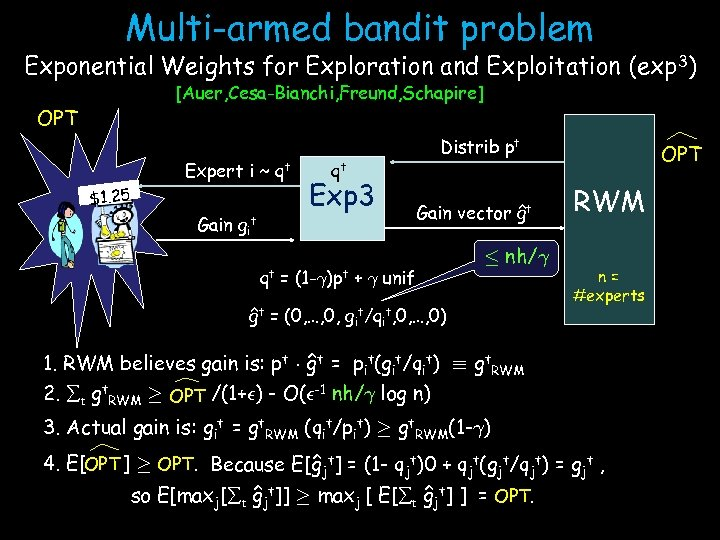Multi-armed bandit problem Exponential Weights for Exploration and Exploitation (exp 3) [Auer, Cesa-Bianchi, Freund, Schapire] OPT Expert i ~ \$1. 25 Gain git qt qt Exp 3 Distrib pt Gain vector ĝt qt = (1 -°)pt + ° unif · nh/° ĝt = (0, …, 0, git/qit, 0, …, 0) OPT RWM n= #experts 1. RWM believes gain is: pt ¢ ĝt = pit(git/qit) ´ gt. RWM 2. t gt. RWM ¸ OPT /(1+²) - O(²-1 nh/° log n) 3. Actual gain is: git = gt. RWM (qit/pit) ¸ gt. RWM(1 -°) 4. E[OPT ] ¸ OPT. Because E[ĝjt] = (1 - qjt)0 + qjt(gjt/qjt) = gjt , so E[maxj[ t ĝjt]] ¸ maxj [ E[ t ĝjt] ] = OPT.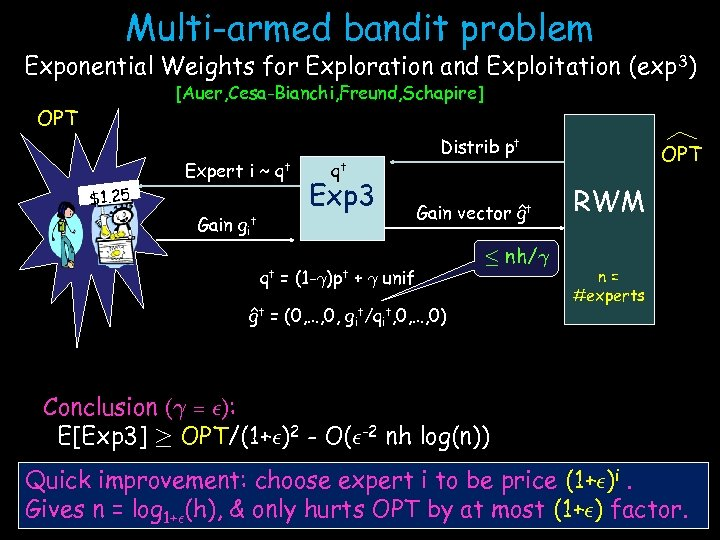Multi-armed bandit problem Exponential Weights for Exploration and Exploitation (exp 3) [Auer, Cesa-Bianchi, Freund, Schapire] OPT Expert i ~ \$1. 25 Gain git qt qt Exp 3 Distrib pt Gain vector ĝt qt = (1 -°)pt + ° unif · nh/° ĝt = (0, …, 0, git/qit, 0, …, 0) OPT RWM n= #experts Conclusion (° = ²): E[Exp 3] ¸ OPT/(1+²)2 - O(²-2 nh log(n)) Quick improvement: choose expert i to be price (1+²)i. Gives n = log 1+²(h), & only hurts OPT by at most (1+²) factor.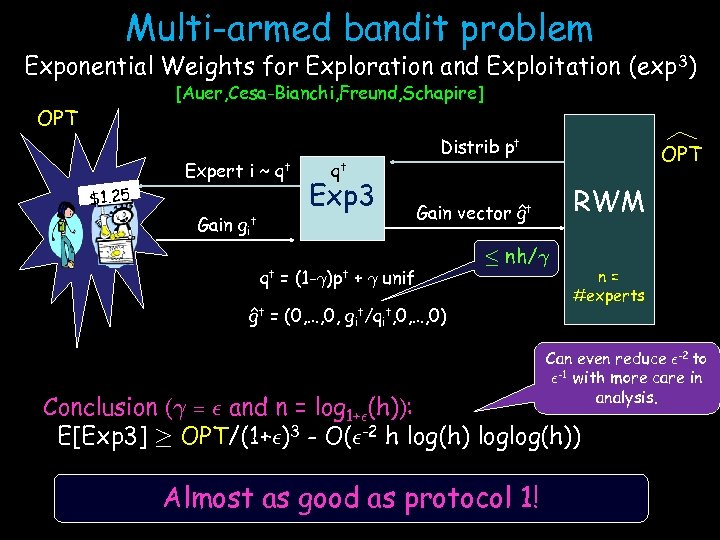Multi-armed bandit problem Exponential Weights for Exploration and Exploitation (exp 3) [Auer, Cesa-Bianchi, Freund, Schapire] OPT Expert i ~ \$1. 25 Gain git qt qt Exp 3 Distrib pt OPT RWM Gain vector ĝt qt = (1 -°)pt + ° unif · nh/° ĝt = (0, …, 0, git/qit, 0, …, 0) n= #experts Can even reduce ²-2 to ²-1 with more care in analysis. Conclusion (° = ² and n = log 1+²(h)): E[Exp 3] ¸ OPT/(1+²)3 - O(²-2 h log(h) loglog(h)) Almost as good as protocol 1!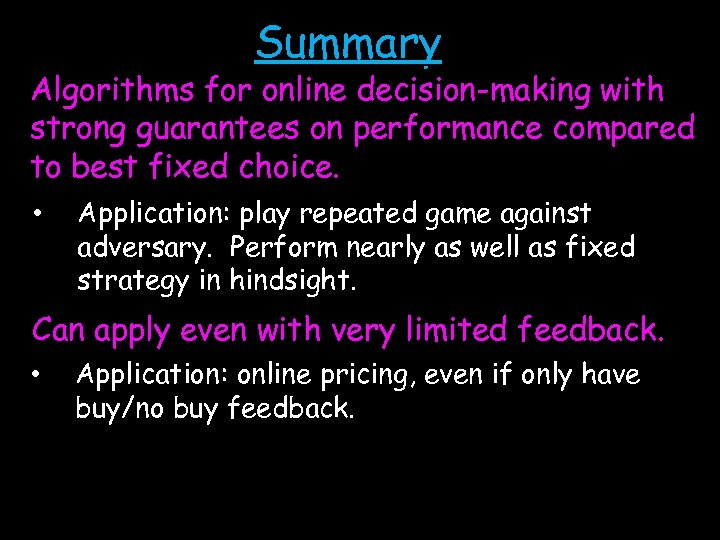Summary Algorithms for online decision-making with strong guarantees on performance compared to best fixed choice. • Application: play repeated game against adversary. Perform nearly as well as fixed strategy in hindsight. Can apply even with very limited feedback. • Application: online pricing, even if only have buy/no buy feedback.